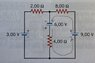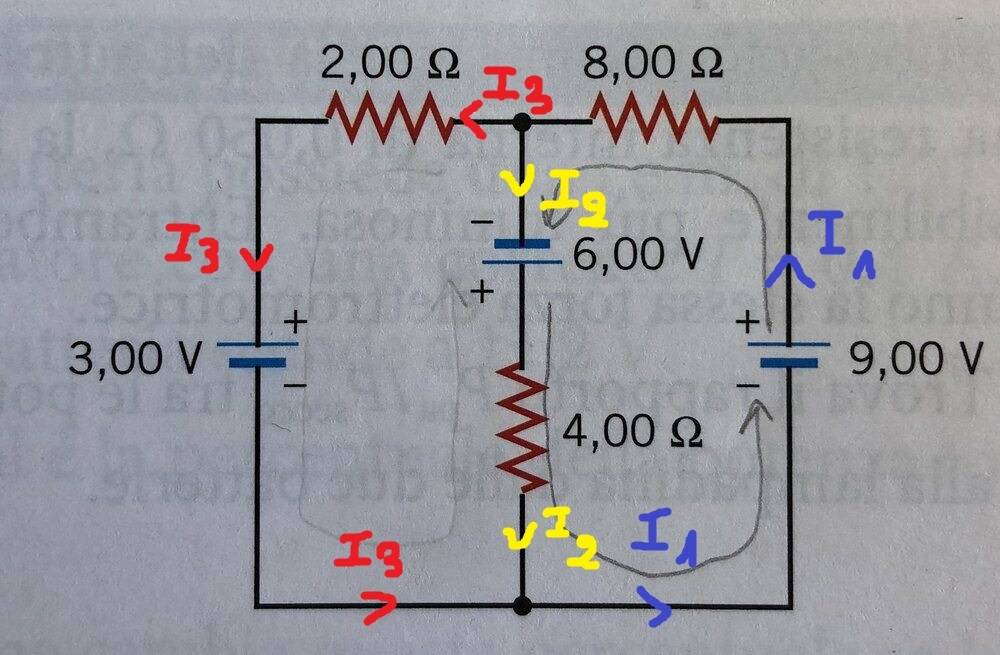# Current in a resistor with Kirchhoff's laws

Gold Member
Homework Statement:
Determine the intensity and direction of the current passing through the resistor of ##4.00\Omega##

(Diagram below)
Relevant Equations:
##I=\frac{V}{R}##
##\Delta\_{Vtot}=0##So, I started by writing down conditions imposed by Kirchhoff's laws, coming up with a system of 3 equations with the 3 unknown currents:
$$\left\{\begin{matrix} 9V-8\Omega I_{8}+6V-4\Omega I_{4}=0 \\ 6V-4\Omega I_{4}+3V-2\Omega I_{2}=0\\ I_{2}+I_{8}=I_{4} \end{matrix}\right.$$
But something's wrong.

Mentor
What makes you think that something's wrong? What result does that system of equations give you for ##I_4## ?

Gold Member
What makes you think that something's wrong? What result does that system of equations give you for ##I_4## ?
That's curious, but re-calculating the same system, the result is now correct😅... but I still have a few questions:
-how do I determine the direction of the current?
-in this particular case, analyzing the two meshes separately, is it totally arbitrary the direction which I make the current flow, for applying Kirchhoff?
-could current flow in opposite directions in the two meshes?

Mentor
That's curious, but re-calculating the same system, the result is now correct😅... but I still have a few questions:
-how do I determine the direction of the current?
-in this particular case, analyzing the two meshes separately, is it totally arbitrary the direction which I make the current flow, for applying Kirchhoff?
-could current flow in opposite directions in the two meshes?
When you initially wrote your equations you made assumptions about the current directions. It's okay to choose their directions arbitrarily so long as you stick to your choices for all subsequent equation writing. The math will automatically sort out the correct directions by giving you the appropriate sign on the result. A negative value tells you that your initial guess was "wrong". Note that there is really no "wrong" choice, as the math will sort itself out!

Yes, the initial choices of current directions is arbitrary. But you must stick to your choices for generating all the equations so that the math is consistent. By the way, you did a loop analysis, not a mesh analysis. Mesh analysis would have employed just two currents for the entire circuit.

You can choose currents to flow in opposite directions for mesh analysis, but you will find that there are certain advantages to choosing them all to circulate in the same direction. One major advantage is that you can write down the equation matrix by inspection with a very simple algorithm. I'm sure you'll soon cover this in your class.

•greg_rack
archaic
I like to choose the battery with the greatest potential and draw a current coming out of it (the + side). Then, at each junction, I draw the necessary new currents, or collapse them into the one they separated from (depending on the junction).Gold Member
When you initially wrote your equations you made assumptions about the current directions. It's okay to choose their directions arbitrarily so long as you stick to your choices for all subsequent equation writing. The math will automatically sort out the correct directions by giving you the appropriate sign on the result. A negative value tells you that your initial guess was "wrong". Note that there is really no "wrong" choice, as the math will sort itself out!

Yes, the initial choices of current directions is arbitrary. But you must stick to your choices for generating all the equations so that the math is consistent. By the way, you did a loop analysis, not a mesh analysis. Mesh analysis would have employed just two currents for the entire circuit.

You can choose currents to flow in opposite directions for mesh analysis, but you will find that there are certain advantages to choosing them all to circulate in the same direction. One major advantage is that you can write down the equation matrix by inspection with a very simple algorithm. I'm sure you'll soon cover this in your class.
Cool! Didn't know what I was doing was a loop analysis :)
So even the third(in my case) equation sticks to what you've said? Independently on the direction chosen, all the math will sort out the correct direction even for the first Kirchhoff's law about input and out current in a node?
I have the impression that, whilst the direction of the first two equations can be arbitrary, for the third one you must be right with choosing the right sum... or don't you?

EDIT: I can't get the same(correct) result by changing directions of the two initial currents. Why?

Last edited:
Gold Member
I like to choose the battery with the greatest potential and draw a current coming out of it (the + side). Then, at each junction, I draw the necessary new currents, or collapse them into the one they separated from (depending on the junction).
View attachment 271384
Got it, and does this have a positive impact on the math?

archaic
Got it, and does this have a positive impact on the math?
No, not really. It's just personal preference.•greg_rack
Mentor
Cool! Didn't know what I was doing was a loop analysis :)
So even the third(in my case) equation sticks to what you've said? Independently on the direction chosen, all the math will sort out the correct direction even for the first Kirchhoff's law about input and out current in a node?
Yes. As long as you stick to your chosen current directions when you write your equations the math will sort itself out, every time.
I have the impression that, whilst the direction of the first two equations can be arbitrary, for the third one you must be right with choosing the right sum... or don't you?

EDIT: I can't get the same(correct) result by changing directions of the two initial currents. Why?
Your KCL sum must reflect your current direction choices. If you change your current directions, you need to write the KCL sum accordingly.

•greg_rack